# Algebra 1 : How to find percentage equivalent to a decimal

## Example Questions

1 2 3 5 Next →

### Example Question #41 : How To Find Percentage Equivalent To A Decimal

Rewrite the following decimal as a percent.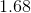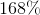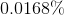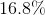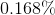Explanation:

Rewrite the following decimal as a percent.To convert between decimals and percents, we simply need to move the decimal point.

When going from a decimal to a percent, move the point two spaces to the right.

When going from a percent to a decimal, move the point two spaces to the left.

So this,Becomes### Example Question #42 : How To Find Percentage Equivalent To A Decimal

Convert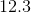to a percentage.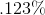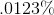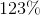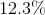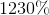Explanation:

To convert a decimal (or whole number) to a percentage, take the decimal and multiply it by 100.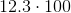### Example Question #43 : How To Find Percentage Equivalent To A Decimal

Convert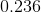to a percentage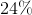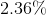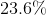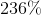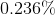Explanation:

To convert any decimal to a percentage, take the number and multiply it by 100. This is the same concept as moving the decimal point two places to the right.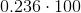This is all you need to do to convert it.

### Example Question #44 : How To Find Percentage Equivalent To A Decimal

Express the decimal as a percent.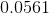0.000561%

5.61%

56.1%

0.561%

5.61%

Explanation:

To find the percent, we take the decimal and multiply it by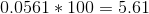The answer is then the equivalent percent.

5.61%

### Example Question #45 : How To Find Percentage Equivalent To A Decimal

Express the decimal as a percentage.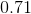7.1%

71%

0.0071%

0.071%

71%

Explanation:

To find the percent, we take the decimal and multiply it by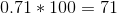The answer is then the equivalent percent.

71%

1 2 3 5 Next →